#### Lattice Vibration, Physics tutorial

Introduction:

A significant feature of the study of solid state physics is the lattice dynamics that concerns itself by the vibrations of atoms regarding their equilibrium sites in a solid. Such vibrations take place at any temperature, even at absolute zero. They are responsible for the thermal properties - heat capacity, thermal conductivity, thermal expansion and so on of insulators and contribute the greater portion of the heat capacity of metals.

Definition:

The Lattice vibration is a continuing periodic oscillation relative to the fixed reference point, or a single complete oscillation.

One-dimensional monatomic lattice:

Let's take a one-dimensional crystal lattice and suppose that the forces between the atoms in this lattice are proportional to the relative displacements from the equilibrium positions. This is termed as the harmonic approximation that holds well provided that the displacements are small. One might think regarding the atoms in the lattice as interconnected through elastic springs.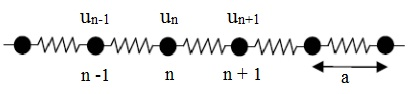Thus, the force applied on nth atom in the lattice is represented by:

Fn = C (un+1 - un) + C (Un-1 - un)

Here, 'C' is the interatomic force (that is, elastic) constant. On applying the Newton's second law to the motion of the n-th atom we get:

M (d2un/dt2) = Fn = C (un+1 - un) + C (un-1 - un) = - C (2un - un+1 - un-1)

Here 'M' is the mass of an atom. Note that we avoided here the interaction of the n-th atom by all however its nearest neighbors. An identical equation must be written for each and every atom in the lattice, resultant in N coupled differential equations, which must be solved concurrently ('N'  is the total number of atoms in the lattice). Additionally, the boundary conditions applied to the end atom in the lattice must be taken into account. Now let us try a solution of the form:

Un = Aei(qxn - ωt)

Here, xn is the equilibrium position of the n-th atom in such a way that xn = na. This equation symbolizes a traveling wave, in which all the atoms oscillate by the same frequency 'ω' and the similar amplitude A and encompass wave vector 'q'. Note that a solution of the form of the above equation is only possible due to the transnational symmetry of the lattice. Now replacing the above equation to the previous equation and canceling the common quantities (that is, the amplitude and the time-dependent factor) we get:

M (-ω2) eiqna = -C [2eiqna - e iq(n+1)a  - e iq(n-1)a]

The above equation can be further simplified by canceling the common factor eiqna that leads to:

2 = C(2 - eiqa - e-iqa) = 2C(1 - cos qa) = 4C sin2 (qa/2)

We thus find out the dispersion relation for the frequency:

ω = √(4C/M) |sin (qa/2)|

That is the relationship between the frequency of vibrations and the wave vector 'q'. This dispersion relation consists of a number of significant properties.

1) On reducing to the first Brillouin zone: The frequency and the displacement of the atoms don't change if we change q by q+2π/a. This signifies that these solutions are physically similar. This lets us to set the range of independent values of 'q' within the first Brillouin zone, that is,

-π/a ≤ q ≤ π/a

Within the range of 'q', the ω versus q is represented in the figure shown below. The maximum frequency is √(4C/M). The frequency is symmetric with respect to the sign change in 'q', that is, ω(q) = ω(-q). This is not surprising as a mode having positive 'q' corresponds to the wave traveling in the lattice from the left to the right and a mode having a negative 'q' corresponds to the wave traveling from the right to the left. As such two directions are equivalent in the lattice the frequency doesn't change with the sign change in 'q'. At the boundaries of the Brillouin zone q = ± (π/a) the solution symbolizes a standing wave Un = A(-1)n e-iωt: atoms oscillate in the opposite phases based on whether 'n' is even or odd. The wave moves neither right nor left.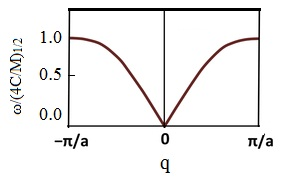2) Phase and group velocity: The phase velocity is stated by:

vp = ω/q

And the group velocity:

vg = dω/dq

The physical difference between the two velocities is that vp is the velocity of the propagation of the plane wave, while vg is the velocity of the propagation of the wave packet. The subsequent is the velocity for the propagation of energy in the medium. For the specific dispersion relation, the equation ω = √(4C/M) |sin (qa/2)| the group velocity is represented by:

vg = √(ca2/M) cos (qa/2)

As is seen from the above equation the group velocity is zero at the edge of the zone where q = ± π/a. In this, the wave is standing and thus the transmission velocity for the energy is zero.

3) Long wavelength limit: The long wavelength limit means that λ >> a. In this limit qa << 1. We can then expand the sine in the equation ω = √(4C/M) |sin (qa/2)| and obtain for the positive frequencies:

ω = √(C/M) qa

We observe that the frequency of vibration is proportional to the wave vector. This is equivalent to the statement that velocity is independent of frequency. In this case:

vp = ω/q = √(C/M)a

It is the velocity of sound for the one dimensional lattice that is consistent having the expression we get earlier for elastic waves.

Diatomic one-dimensional lattice:

Now we take a one-dimensional lattice having two non-equivalent atoms in the unit cell. It appears that the diatomic lattice represent significant characteristics different from the monoatomic case. The figure below represents a diatomic lattice having the unit cell composed of two atoms of masses M1 and M2 by the distance between the two neighboring atoms 'a'.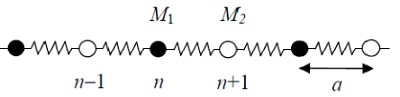We can treat the motion of this lattice in an identical fashion as for monoatomic lattice. Though, in this case because we have two different types of atoms, we must write two equations of motion:

M1 (d2un/dt2) = - C (2un - un+1 - un-1)

M2 (d2un+1/dt2) = - C (2un+1 - un+2 - un)

In analogy having the monoatomic lattice we are looking for the solution in the form of traveling mode for the two atoms: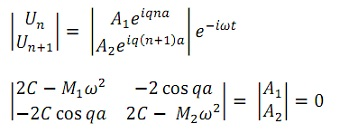This is the system of linear homogeneous equations for the unknowns A1 and A2. A non-trivial solution exists only when the determinant of the matrix is zero. This leads to the secular equation:

(2C - M1ω2) (2C - M2ω2) - 4C2 cos2 qa = 0

ω2 = C [(1/M1) + (1/M2)] ± C√(1/M1 + 1/M2)2 - (4sin2qa/M1M2)

Based on the sign in this formula there are two different solutions corresponding to two various dispersion curves, as is shown in the figure given below: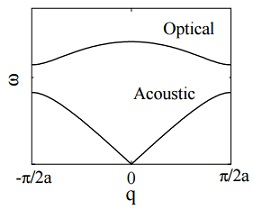The lower curve is termed as the acoustic branch, whereas the upper curve is termed as the optical branch. The optical branch begins at q = 0 and ω = 0. Then by increasing 'q' the frequency rises in a linear fashion. This is why this branch is termed as acoustic: it corresponds to elastic waves or sound. Ultimately this curve saturates at the edge of the Brillouin zone. On the other hand, the optical branch consists of a non-zero frequency at zero 'q'.

ωo = √[2C(1/M1 + 1/M2)]

And it doesn't change much by 'q'.

The difference between the acoustic and optical branches of lattice vibrations can be seen most clearly through comparing them at q = 0 (that is, infinite wavelength). As follows from the equation, for the acoustic branch ω = 0 and A1 = A2. Therefore, in this limit the two atoms in the cell have the similar amplitude and the phase. Thus, the molecule oscillates as a rigid body, as shown in the figure below for the acoustic mode. On the other hand, for optical vibrations, replacing the equation √ [2C (1/M1 + 1/M2)], we get for q = 0:

M1A1 + M2A2 = 0

This means that the optical oscillation occurs in such a manner that the center of mass of a molecule remains fixed. The two atoms move in out of phase as shown in figure below. The frequency of such vibrations lies in infrared region that is the reason for referring to this branch as optical.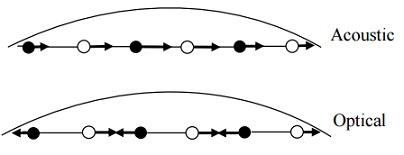Three-dimension:

The theory of the division of the vibrational modes into the acoustic and optical branches can be generalized to be applicable to (3-D) three-dimensional structure. To avoid the mathematical details we shall present simply a qualitative discussion. Let's take, first, the monatomic Bravais lattice, in which each and every unit cell consists of a single atom. The solution in three dimensions can be represented in terms of the normal modes as:

Un = Ae i(qr - ωt)

Here the wave vector 'q' specifies both the wavelength and direction of the propagation. The vector 'A' finds out the amplitude and also the direction of vibration of the atoms. Therefore this vector specifies the polarization of the wave, that is, whether the wave is longitudinal (that is, A parallel to q) or transverse (that is, A perpendicular to q). When we replace the above equation into the equation of motion, we get three simultaneous equations comprising Ax, Ay and Az, the components of A. Such equations are coupled altogether and are equivalent to a 3 x 3 matrix equation. The roots of this equation lead to three dissimilar dispersion relations, or three dispersion curves, as shown in the figure below. All the three branches pass via the origin that signifies all the branches are acoustic. This is obviously to be expected, as we are dealing by the monatomic Bravais lattice.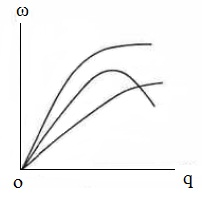The three branches in the figure above differ in their polarization. When 'q' lies all along a direction of high symmetry - for illustration, the  or  directions - these waves might be categorized as either pure longitudinal or pure transverse waves. In that case, two of the branches are transverse and one is longitudinal. One generally refers to these as the TA - transverse acoustic and LA - longitudinal acoustic branches, correspondingly. Though, along non-symmetry directions the waves might not be pure longitudinal or pure transverse, however have a mixed character.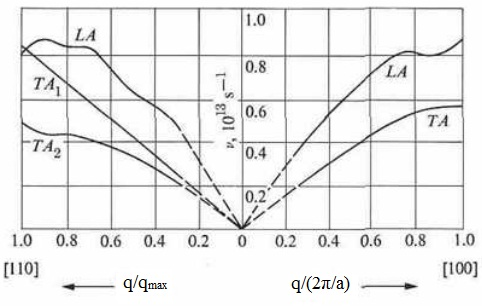The figure represents the dispersion curves for Al in the  and  directions. Note that in some high-symmetry directions, like the  in Al, the two transverse branches coincide. The branches are then stated to be degenerate.

We turn our attention now to the non-Bravais (3-D) three-dimensional lattice. In this, the unit cell includes two or more atoms. When there are 's' atoms per cell, then on the basis of our prior experience we sum up that there are 3s dispersion curves. Of these, three branches are acoustic, and the remaining (3s - 3) are optical. The mathematical justification for this assertion is as follows: We write the equation of motion for each atom in the cell, which results in s equations. Since these are vector equations, they are equivalent to 3s scalar equations, which have 3s roots. It can be shown that three of these roots always vanish at q = 0, which results in three acoustic branches. The remaining (3s - 3) roots, thus, belong to the optical branches, as illustrated above. The acoustic branches might be categorized, as before, by their polarizations as TA1, TA2, and LA. The optical branches can as well be categorized as longitudinal or transverse when 'q' lies all along a high symmetry direction, and one speaks of LO and TO branches. As in one-dimensional case, one can as well illustrate that, for an optical branch, the atoms in the unit cell vibrate out of phase relative to one other. As an illustration of a non-Bravais lattice, the dispersion curves for Ge are shown in the figure given below. As there are two atoms per unit cell in germanium, there are six branches: three acoustic and three optical. It will be noted that the two transverse branches are degenerate all along the  direction, as pointed out earlier.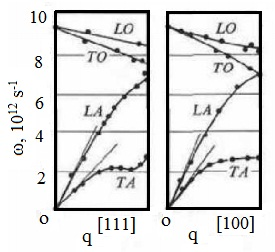Phonons:

Till now illustrated a classical approach to the lattice vibrations. As we are familiar that from quantum mechanics the energy levels of the harmonic oscillator is quantized. Likewise the energy levels of lattice vibrations are quantized. The quantum of vibration is termed as a phonon in analogy with the photon that is the quantum of the electromagnetic wave. We are familiar that the allowed energy levels of the harmonic oscillator are represented by:

E = (n + 1/2)(h/2π)ω

Here 'n' is the quantum number. A normal vibration mode in a crystal of frequency 'ω' is represented by the equation Un = Ae i(qr - ωt). If the energy of this mode is represented by the equation E = (n + 1/2)(h/2π)ω; we can state that this mode is occupied by 'n' phonons of energy (h/2π) ω. The term (h/2π) ω is the zero point energy of the mode.

Let us now formulate a comparison between the classical and quantum solutions in one-dimensional case. Consider a normal vibration:

u = Ae i(qx - ωt)

Here 'u' is the displacement of an atom from its equilibrium position 'x' and 'A' is the amplitude. The energy of this vibrational mode averaged over time is:

E = 1/2 Mω2A2 = (n + 1/2)(h/2π)ω

This is evident from the above equation above that there is a relationship between the amplitude of vibration and the frequency and the phonon occupation of the mode. In the classical mechanics any amplitude of vibration is possible, while in quantum mechanics only discrete values are allowed. This is shown in the figure given below: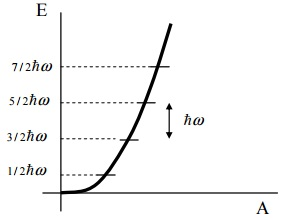The lattice having 's' atoms in a unit cell is illustrated by 3s independent oscillators. The frequencies of the normal modes of these oscillators will be represented by the solution of 3s linear equations as we illustrated before. They are ωp(q), where 'p' signifies a specific mode, that is, p = 1,...3s. The energy of this mode is represented by:

Eqp = (nqp + 1/2) (h/2π) ωp (q)

Here nqp occupation is the number of normal mode and is an integer. A vibrational state of the whole crystal is specified by giving the occupation numbers for each of the 3s modes. The net vibrational energy of the crystal is the sum of the energies of the individual modes, in such a way that:

E = Σqp Eqp = Σqp (nqp + 1/2) (h/2π) ωp (q)

The Phonons can interact by other particles like photons, neutrons and electrons. This interaction takes place such as if photon had a momentum (h/2π) q. Though, a phonon doesn't carry real physical momentum. The main reason is that the center of mass of the crystal doesn't change its position under vibrations (apart from q = 0).  In crystals, there exist selection rules for allowed transitions between the quantum states. We observe that the elastic scattering of an x-ray photon via a crystal is governed through the wave vector selection rule k′ = k + G, here 'G' is a vector in the reciprocal lattice; 'k' is the wave vector of the incident photon and  k′ is the wave vector of the scattered photon. This equation can be taken as condition for the conservation of the momentum of the entire system, in which the lattice obtains a momentum - (h/2π) G.  If the scattering of photon is inelastic and is accompanied through the excitation or absorption of a phonon the selection rule becomes:

K' = k ± q + G

Here sign (+) corresponds to the creation of phonon and sign (-) corresponds to the absorption of phonon. Phonon dispersion relations ωp q can be found out by the inelastic scattering of neutrons with emission or absorption of phonons. In this case in addition to the condition of the momentum conservation we have the necessity of conservation of energy. The latter condition can be represented as: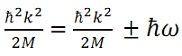Here 'M' is the mass of the neutron and (h/2π) k and (h/2π) k′ are the momenta of the incident and scattered neutron. Once we are familiar in experiment the kinetic energy of the incident and scattered neutrons from the above equation, we can find out the frequency of the emitted or absorbed phonon. Then experimentally we need to find out such directions that characterized through highest intensity of the scattered beam. For such directions the conditions are satisfied and thus from the above equation we can find out the wave vector of the phonon. Thus, this is the way to get the dispersion conditions for the frequency of phonons that we illustrated before.

Tutorsglobe: A way to secure high grade in your curriculum (Online Tutoring)